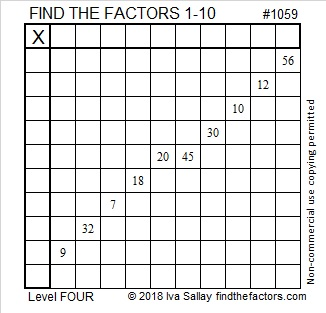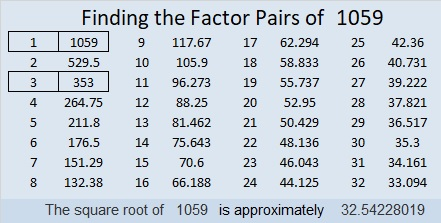# 1059 and Level 4

A level four puzzle is only a little more difficult than a level three puzzle. Instead of starting with the top clue and working down cell by cell, the next clue that you need could be anywhere in the puzzle. It may be a little harder, but you can still solve this puzzle!Print the puzzles or type the solution in this excel file: 10-factors-1054-1062

What can I tell you about the number 1059?

• 1059 is a composite number.
• Prime factorization: 1059 = 3 × 353
• The exponents in the prime factorization are 1 and 1. Adding one to each and multiplying we get (1 + 1)(1 + 1) = 2 × 2 = 4. Therefore 1059 has exactly 4 factors.
• Factors of 1059: 1, 3, 353, 1059
• Factor pairs: 1059 = 1 × 1059 or 3 × 353
• 1059 has no square factors that allow its square root to be simplified. √1059 ≈ 32.542281059 is the hypotenuse of a Pythagorean triple:
675-816-1059 which is 3 times (225-272-353)

1059 is palindrome 636 in BASE 13 because 6(13²) + 3(13) + 6(1) = 1059

This site uses Akismet to reduce spam. Learn how your comment data is processed.